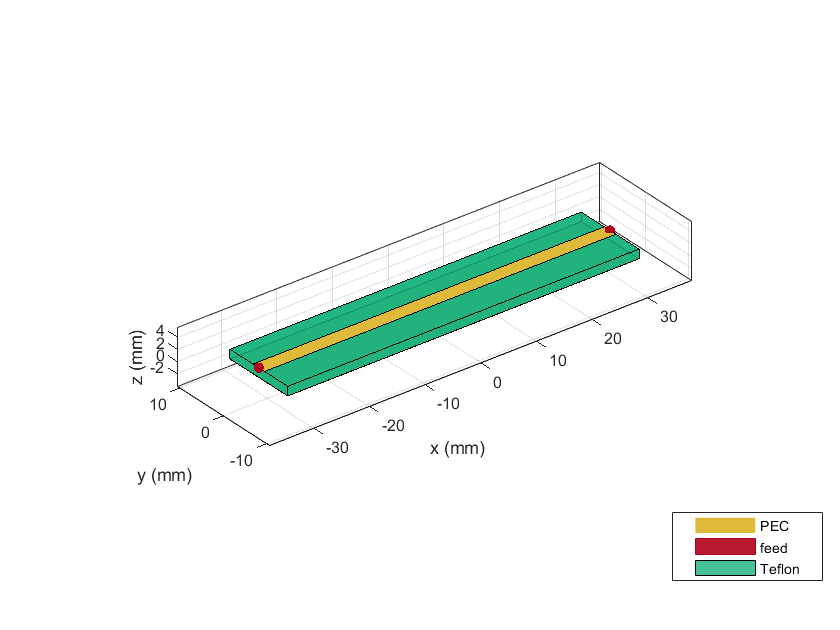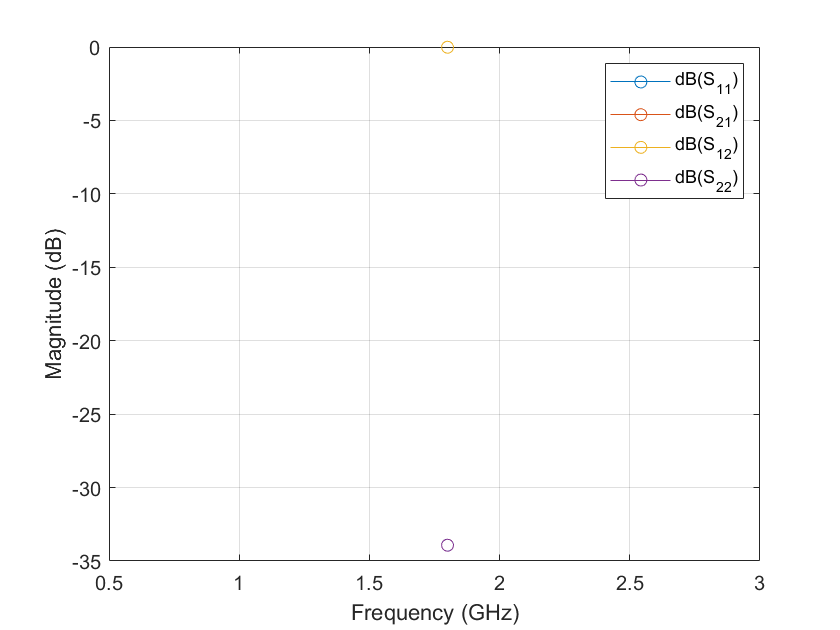# design

Design microstrip transmission line around specified frequency

## Syntax

``mline = design(mlineobj,frequency)``
``mline = design(___,Name=Value)``

## Description

````mline = design(mlineobj,frequency)` designs a microstrip transmission line around the specified frequency.```

example

````mline = design(___,Name=Value)` designs a microstrip transmission line with additional options specified using name-value arguments. NotePCB components designed using the `design` function operate around the specified frequency with a 10-15% tolerance. ```

## Examples

collapse all

Design a microstrip line around 1.8 GHz and with a characteristic impedance of 75 ohms.

```mline = design(microstripLine,1.8e9,Z0=75); figure; show(mline); ```Plot the S-parameters of this line.

```spar = sparameters(mline,1.8e9); rfplot(spar)```## Input Arguments

collapse all

Microstrip transmission line, specified as a `microstripLine` object.

Example: `mline = microstripLine; design(mline,2e9)` designs a microstrip transmission line around a frequency of 2 GHz.

Design frequency of the transmission line, specified as a real positive scalar in hertz.

Example: `55e6`

Data Types: `double`

### Name-Value Arguments

Example: `Z0=70`

Characteristic impedance of the microstrip transmission line, specified as a positive scalar in ohms.

Data Types: `double`

Length of the line, specified as a positive scalar in Lambda.

Data Types: `double`

## Output Arguments

collapse all

Microstrip transmission line operating around the specified frequency, returned as a `microstripLine` object.

## Version History

Introduced in R2021b